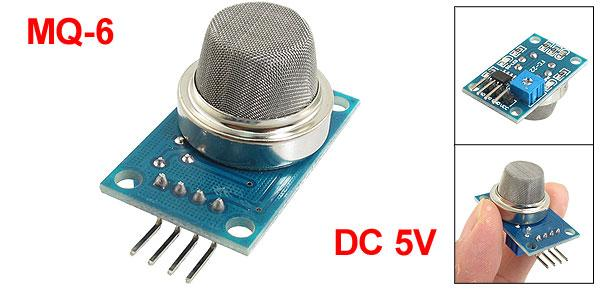# Wemos and an MQ6 Gas Sensor

The MQ6 Gas Sensor module is useful for gas leakage detection. They are used in gas leakage detecting equipments in home and industry, are suitable for detecting of LPG, iso-butane, propane, LNG, avoid the noise of alcohol , cooking fumes and cigarette smoke.  Due to its high sensitivity and response time, measurements can be taken as soon as possible.

The sensitivity of the sensor can be adjusted by using the potentiometer.FEATURES

* High sensitivity to LPG, iso-butane, propane
* Small sensitivity to alcohol, smoke.
* Fast response .
* Stable and long life
* Simple drive circuit

Connection

here are the connections for my sensor

Wemos Gas Sensor
3v3 VCC
GND GND
NC DO
Analog A0 AO

Code

[codesyntax lang=”cpp”]

```void setup()
{
Serial.begin(9600);
}

void loop()
{
float sensorVoltage;
float sensorValue;

sensorVoltage = sensorValue/1024*5.0;

Serial.print("sensor voltage = ");
Serial.print(sensorVoltage);
Serial.println(" V");
delay(1000);
}```

[/codesyntax]

Results

Again look at the serial monitor, in this case I noticed that it took about 3 minutes for the readings to stabilise, something you may want to factor in, the heater of the sensor does take time to heat up, in fact some sources on the internet suggest 24 hours

sensor voltage = 1.14 V
sensor voltage = 1.44 V
sensor voltage = 1.71 V
sensor voltage = 1.95 V
sensor voltage = 2.16 V
sensor voltage = 2.32 V
sensor voltage = 2.46 V
sensor voltage = 2.55 V
sensor voltage = 2.56 V
sensor voltage = 2.47 V
sensor voltage = 2.28 V
sensor voltage = 2.08 V
sensor voltage = 1.90 V
sensor voltage = 1.76 V
sensor voltage = 1.63 V
sensor voltage = 1.52 V
sensor voltage = 1.43 V
sensor voltage = 1.34 V
sensor voltage = 1.26 V

sensor voltage = 0.40 V
sensor voltage = 0.40 V
sensor voltage = 0.40 V
sensor voltage = 0.39 V
sensor voltage = 0.39 V
sensor voltage = 0.39 V
sensor voltage = 0.39 V